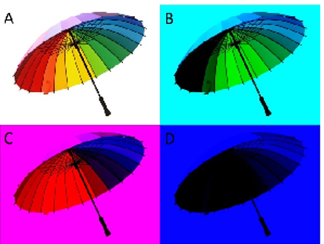##### KAPEC GEOTASK?
• The learning process is entertaining and exciting
• The solved tasks turn into coordinates
• The chance to follow your progress
• Along with your schoolmates and friends, go on an adventure, searching for hideouts
• E-mail:

# 115.2. Light spectre and colour formation.

#### Photograph edited picture A and in result got picture B,C and D. Which amount of colour has to be decreased till minimum to get picture D?#### Next task: 491. Light spectre and colour formation.

Grade:
8
Subject
Physics
Theme
Light and colours
Subtheme
Light spectre and colour formation.

#### Solve tasks to find hidden Geo point coordinates

1. Algebra: Linear function research.
2. Geometry: Polygon angle sum, convex or concaved quadrangle, polygon diagonale.
3. Algebra: Rational numbers.
4. Chemistry: Waters physical properties. Solution (part of weight).
5. Chemistry: Substances multiplicity.
6. Algebra: Fraction multiplication and division.
7. Geometry: Parallelogram, diamond, triangle, right-angled triangle, trapeze area.
8. Algebra: Average arithmetical
9. Physics: Archimedes law.
10. Algebra: Linear equalities and inequalities solution.